Search
Document Center

# Alarm - monitor Nginx access logs in real time

Last Updated: Jan 30, 2018

Currently, Log Service supports saving a query statement as Saved Search, setting a trigger cycle (interval) for query, setting judgment conditions for execution results, and sending alarms. You can also set alarm actions, namely, the way to inform you when the result of regularly running Saved Search triggers alarm conditions.

Currently the following 3 notification methods are supported:

• Notification center: Multiple contacts can be set in Alibaba Cloud notification center. Notifications are sent by using emails and text messages.
• WebHook: Including DingTalk robot and custom WebHook.

For more information about alarm function configuration and use, see Set alarm rules. In addition to monitoring and alarming by using Log Service, you can also use Alibaba Cloud CloudMonitor to monitor the metrics of Log Service. Notification messages are sent to you when alarm conditions are triggered.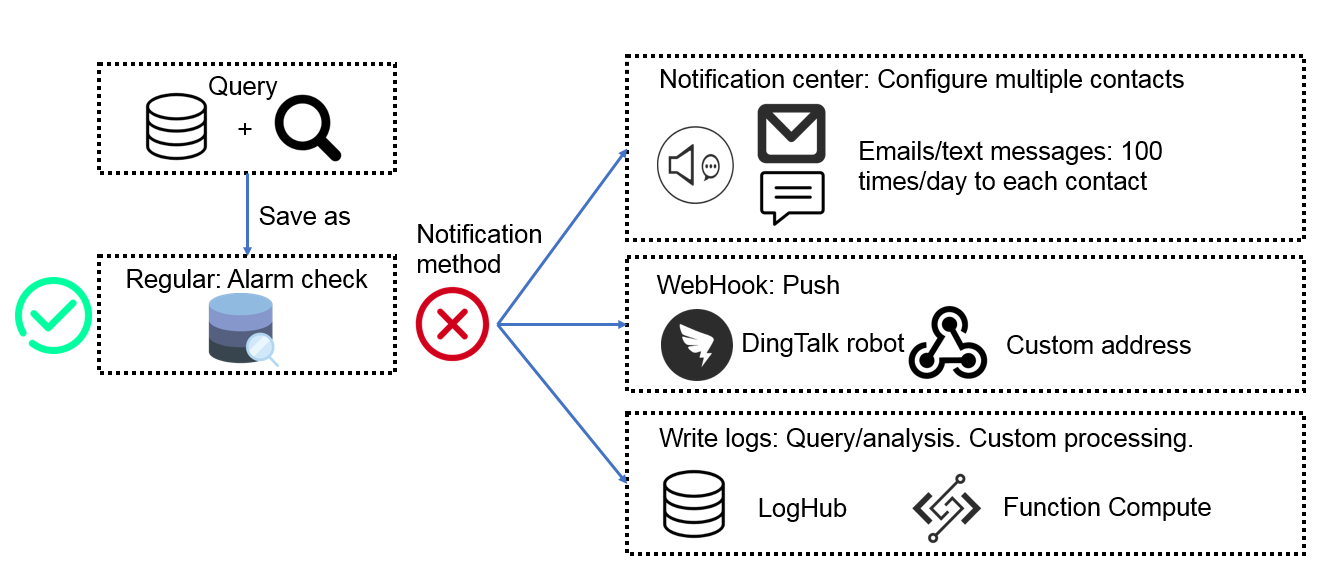## Scenarios

Taking Nginx logs as an example, we demonstrate how to use Log Service to perform regular query and analysis on collected log information and determine the following business issues based on the LogSearch results:

• Whether or not an error exists.
• Whether or not a performance problem exists.
• Whether or not the traffic has a sudden decrease or increase.

## Preparation (Nginx log access)

1. Collect the log data.

1. Enter the Data Source Access Wizard on the Logstore List page and then select Nginx Access Log.
2. Enter the Configuration Name, Log Path, Nginx Log Format, and Nginx Key Name, and configure the Advanced Settings as per your needs.
3. Click Next to configure the index.
2. Set the index.

For more information, see Index settings and Visualization or Best Practice - Website log analysis case.

3. Set the views and alarms for key metrics.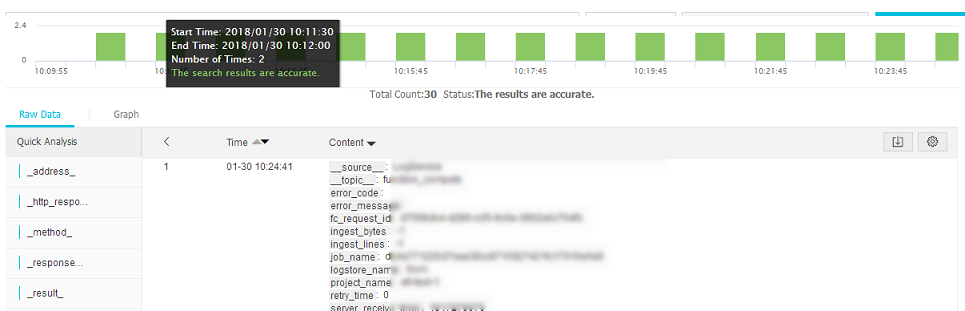Sample views: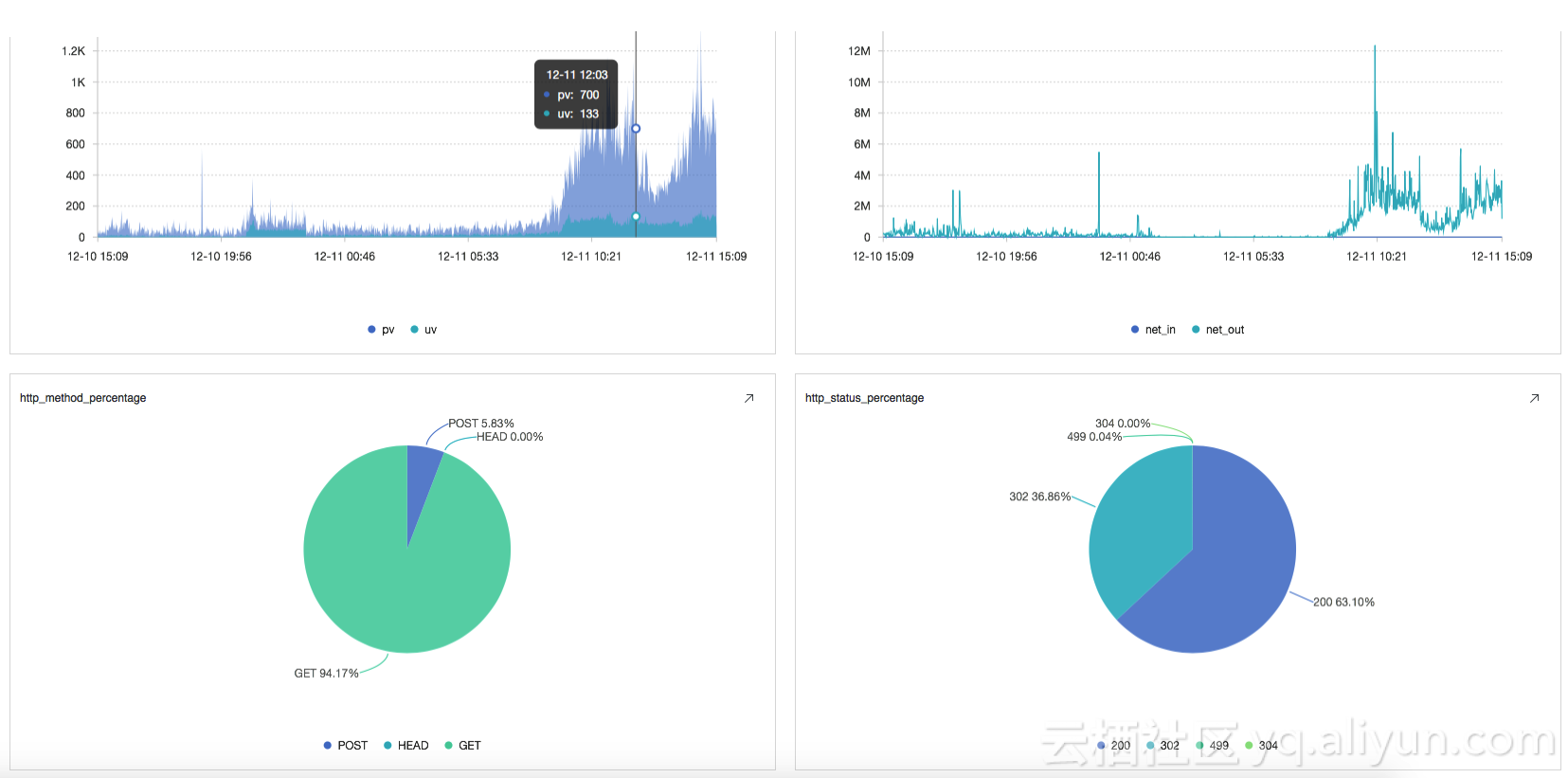## Procedure

### 1. Determine if any error exists

The common errors are as follows: 404 (the request cannot find the address)/502/500 (error on the server). Generally we only focus on 500 (error on the server).

Determine if a 500 error exists. You can use the following query to count the number of errors (c) in the unit time and set the alarm rule as if c > 0, an alarm is to be sent.

``status:500 | select count(1) as c``

This method is relatively simple but too sensitive. For services facing relatively high business pressure, a few 500 errors are common. In response to this situation, you can set the Trigger Count as 2 in the alarm conditions so that alarms are only triggered when the conditions are met for 2 times in a row.

### 2. Determine if any performance problem exists

Although no error occurs in server operation, the latency might be increased. You can set an alarm for latency.

For example, you can calculate all the write request (“Post”) latencies of an interface (“/adduser”) using the following method. Set the alarm rule as l > 300000, which means when the average value exceeds 300 ms, an alarm is to be sent.

``Method:Post and URL:"/adduser" | select avg(Latency) as l``

Alarm sending by using average value is simple and direct, however, this method can average some individual request latencies, which cannot reflect problems. For example, you can compute a mathematical distribution for the latencies of the time period, namely, dividing into 20 intervals and calculating the number in each interval. You can see on the histogram that most of the request latencies are low (< 20 ms), but the highest value is 2.5s.

``Method:Post and URL:"/adduser" | select numeric_histogram(20, Latency)``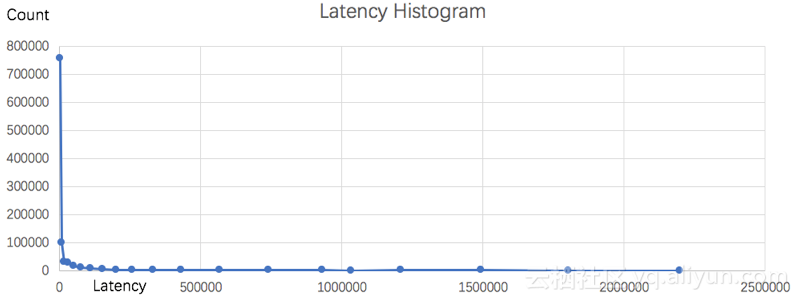To deal with this situation, you can use the percentile in mathematical statistics (the maximum latency is 99%) as the alarm condition. In this way, you can exclude false alarms triggered by accidental high latencies and reflect the overall situation of latencies. The following statements calculate the latency of the 99th percentile, approx_percentile (Latency, 0.99). You can also change the second parameter to calculate the latency of other percentiles, for example, the request latency of the 50th percentile, approx_percentile (Latency,0.5).

``Method:Post and URL:"/adduser" | select approx_percentile(Latency, 0.99) as p99``

In a monitoring scenario, you can chart the average latency, the 50th percentile latency, and the 90th percentile latency. The following is the chart showing the latencies of every minute in a day (1440 minutes):

``* | select avg(Latency) as l, approx_percentile(Latency, 0.5) as p50, approx_percentile(Latency, 0.99) as p99, date_trunc('minute', time) as t group by t order by t desc limit 1440``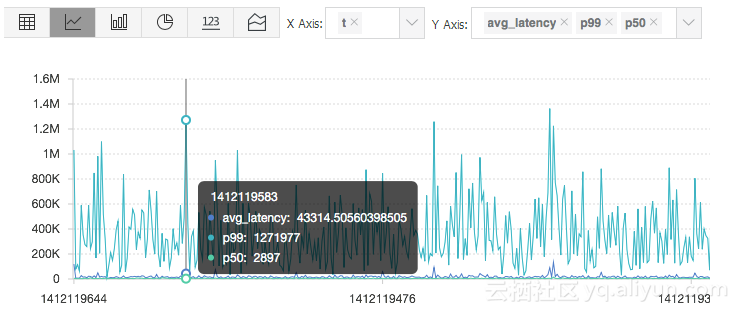### 3. Determine if the traffic has a sudden decrease or increase

The natural traffic on the server is usually in line with probability distribution, which means a process of slow increase or decrease exists. Sudden decrease or increase in traffic indicates great changes in a short period of time, which is usually abnormal and needs special attention.

As shown in the following monitoring chart, the traffic decreases by over 30% within 2 minutes and resumes rapidly within 2 minutes.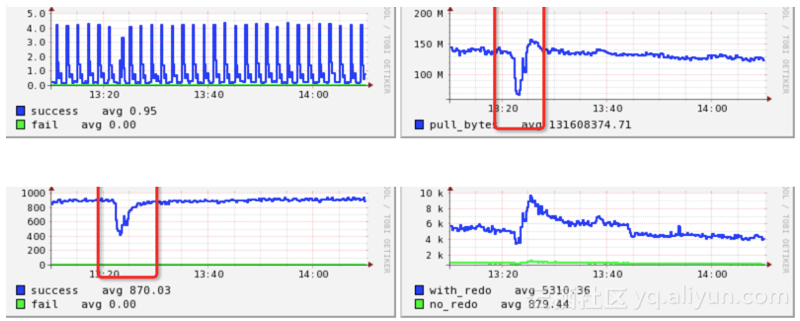Sudden decrease or increase is usually within the following reference frames:

• The previous time window: Link relative ratio compared with the previous time period.
• The window of the same time period of the previous day: Link relative ratio compared with the previous day.
• The window of the same time period of the previous week: Link relative ratio compared with the previous week.

Take the first reference frame as an example in this article to calculate the ratio of change of inflow data. We can also calculate other traffic data such as QPS.

#### 3.1 Define a calculation window

Define a window of 1 minute to count the inflow of this minute. The following is a statistic result with a 5-minute interval.

``* | select sum(inflow)/(max(__time__)-min(__time__)) as inflow , __time__-__time__%60  as window_time from log group by window_time order by window_time limit 15``

We can see from the result distribution that the average inflow in every window `sum(inflow)/(max(__time__)-min(__time__))` is even.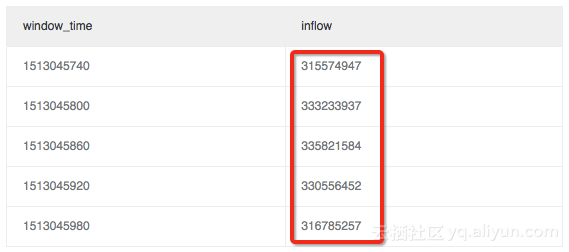#### 3.2 The variance in the calculation window (max_ratio)

Use subquery here. Write a query and calculate the ratio of change between the maximum value or the minimum value and the average value (max_ratio) from the preceding result. For example, in the following calculation result, max_ratio is 1.02. You can define an alarm rule, for example, if max_ratio > 1.5 (the ratio of change exceeds 50%), an alarm is to be sent.

`` * | select max(inflow)/avg(inflow) as max_ratio from (select sum(inflow)/(max(__time__)-min(__time__)) as inflow , __time__-__time__%60  as window_time from log group by window_time order by window_time limit 15)``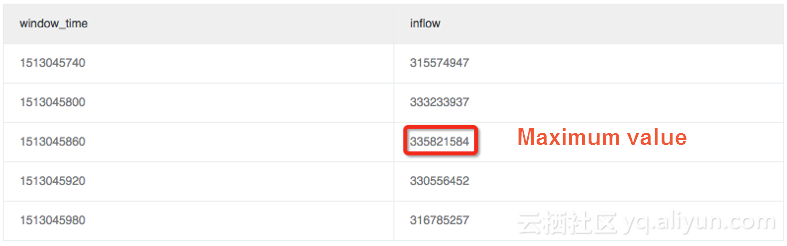#### 3.3 The variance in the calculation window (latest_ratio)

In some scenarios, we pay more attention to the fluctuation of the latest value (whether recovered or not). Determine by getting the inflow in the maximum windows_time with the max_by method. Here in this example, lastest_ratio=0.97.

Note: The function calculation result of max_by is of character type, which must be converted to numeric type. To calculate relative ratio of change, you can replace it with (1.0-max_by(inflow, window_time)/1.0/avg(inflow)) as lastest_ratio.

`` * | select max_by(inflow, window_time)/1.0/avg(inflow) as lastest_ratio from (select sum(inflow)/(max(__time__)-min(__time__)) as inflow , __time__-__time__%60  as window_time from log group by window_time order by window_time limit 15)``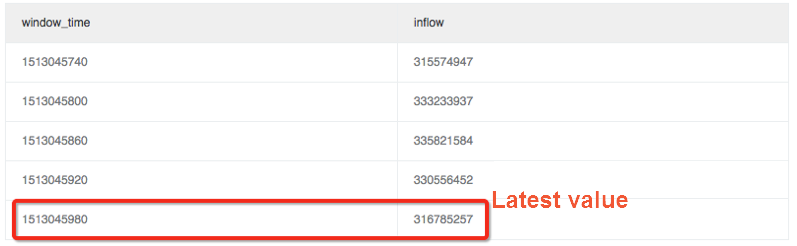#### 3.4 The variance in the calculation window (define the fluctuation ratio, namely, the ratio of change between the current value and the previous value)

Another method for calculating fluctuation ratio is First Derivative in mathematics, namely, the ratio of change between the value of the current window and the value of the previous window.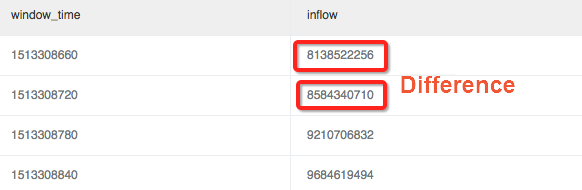Use window function (lag) for calculation. From the window function, extract the current inflow and the inflow of the previous period to calculate the difference by using `lag(inflow, 1, inflow)over()` and divide the calculated difference value by the current value to get the ratio of change:

`` * | select (inflow- lag(inflow, 1, inflow)over() )*1.0/inflow as diff, from_unixtime(window_time) from (select sum(inflow)/(max(__time__)-min(__time__)) as inflow , __time__-__time__%60  as window_time from log group by window_time order by window_time limit 15)``

In the example, a relatively major decrease occurs in traffic at 11:39 (the ratio of change between windows exceeds 40%):

To define an absolute ratio of change, you can use abs function (absolute value) to unify the calculation result.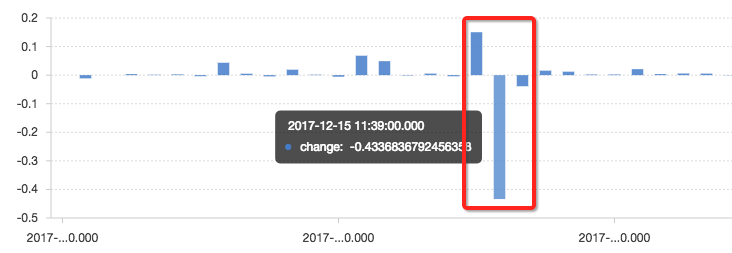## Conclusion

The query and analysis function of Log Service is complete SQL92, which supports various mathematical statistics and computing. Anyone who can use SQL can perform fast analysis. Have a try!